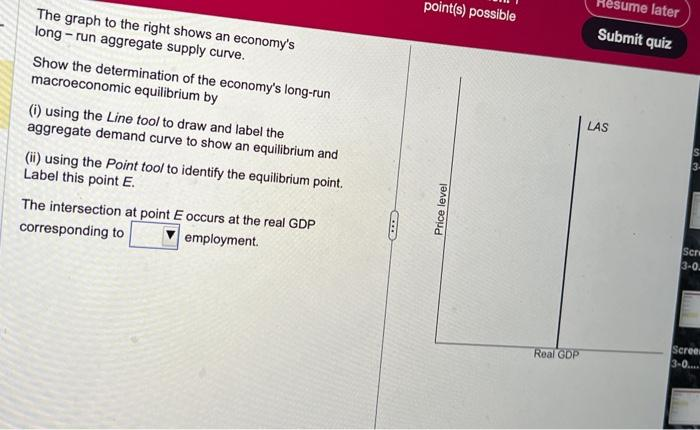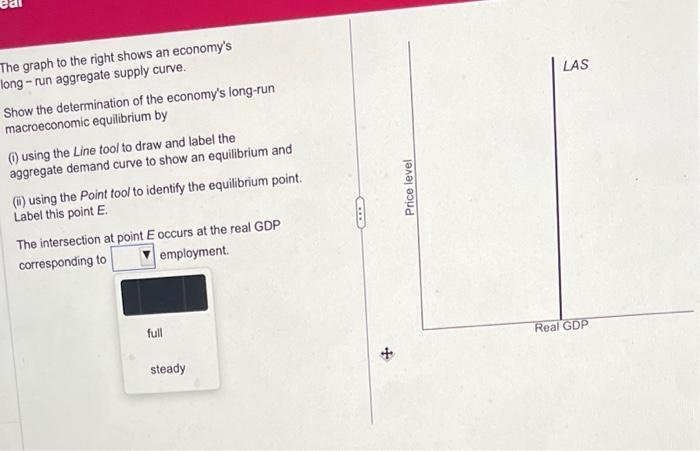# Question The graph to the right shows an economy's long - run aggregate supply curve. Show the determination of the economy's long-run macroeconomic equilibrium by (i) using the Line tool to draw and label the aggregate demand curve to show an equilibrium and (ii) using the Point tool to identify the equilibrium point. Label this point \( E \). The intersection at point \( E \) occurs at the real GDP corresponding to employment. The graph to the right shows an economy's long - run aggregate supply curve. Show the determination of the economy's long-run macroeconomic equilibrium by (i) using the Line tool to draw and label the aggregate demand curve to show an equilibrium and (ii) using the Point tool to identify the equilibrium point. Label this point \( E \). The intersection at point E occurs at the real GDP corresponding to employment.Transcribed Image Text: The graph to the right shows an economy's long - run aggregate supply curve. Show the determination of the economy's long-run macroeconomic equilibrium by (i) using the Line tool to draw and label the aggregate demand curve to show an equilibrium and (ii) using the Point tool to identify the equilibrium point. Label this point \( E \). The intersection at point \( E \) occurs at the real GDP corresponding to employment. The graph to the right shows an economy's long - run aggregate supply curve. Show the determination of the economy's long-run macroeconomic equilibrium by (i) using the Line tool to draw and label the aggregate demand curve to show an equilibrium and (ii) using the Point tool to identify the equilibrium point. Label this point \( E \). The intersection at point E occurs at the real GDP corresponding to employment.
More
Transcribed Image Text: The graph to the right shows an economy's long - run aggregate supply curve. Show the determination of the economy's long-run macroeconomic equilibrium by (i) using the Line tool to draw and label the aggregate demand curve to show an equilibrium and (ii) using the Point tool to identify the equilibrium point. Label this point \( E \). The intersection at point \( E \) occurs at the real GDP corresponding to employment. The graph to the right shows an economy's long - run aggregate supply curve. Show the determination of the economy's long-run macroeconomic equilibrium by (i) using the Line tool to draw and label the aggregate demand curve to show an equilibrium and (ii) using the Point tool to identify the equilibrium point. Label this point \( E \). The intersection at point E occurs at the real GDP corresponding to employment.